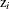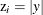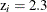The MCMC Procedure

Assignments of Parameters

In general, you cannot alter the values of any model parameters in PROC MCMC. For example, the following assignment statement produces an error:

```parms alpha;
alpha = 27;
```

This restriction prevents incorrect calculation of the posterior density—assignments of parameters in the program would override the parameter values generated by PROC MCMC and lead to an incorrect value of the density function.

However, you can modify parameter values and assign initial values to parameters within the block defined by the BEGINCNST and ENDCNST statements. The following syntax is allowed:

```parms alpha;
begincnst;
alpha = 27;
endcnst;
```

The initial value of `alpha` is 27. Assignments within the BEGINCNST/ENDCNST block override initial values specified in the PARMS statement. For example, with the following statements, the Markov chain starts at `alpha` = 27, not 23.

```parms alpha 23;
begincnst;
alpha = 27;
endcnst;
```

This feature enables you to systematically assign initial values. Suppose that `z` is an array parameter of the same length as the number of observations in the input data set. You want to start the Markov chain with eachhaving a different value depending on the data set variable `y`. The following statements setfor the first half of the observations andfor the rest:

```/* a rather artificial input data set. */
data inputdata;
do ind = 1 to 10;
y = rand('normal');
output;
end;
run;

proc mcmc data=inputdata;
array z;
begincnst;
if ind <= 5 then z[ind] = abs(y);
else z[ind] = 2.3;
endcnst;
parms z:;
prior z: ~ normal(0, sd=1);
model general(0);
run;
```

Elements of `z` are modified as PROC MCMC executes the programming statements between the BEGINCNST and ENDCNST statements. This feature could be useful when you use the GENERAL function and you find that the PARMS statements are too cumbersome for assigning starting values.